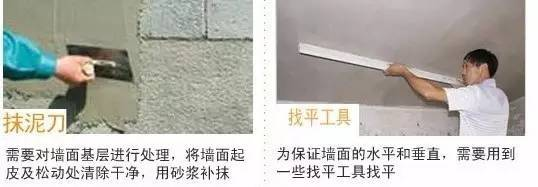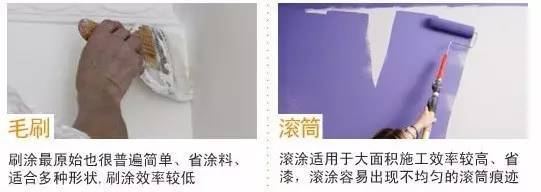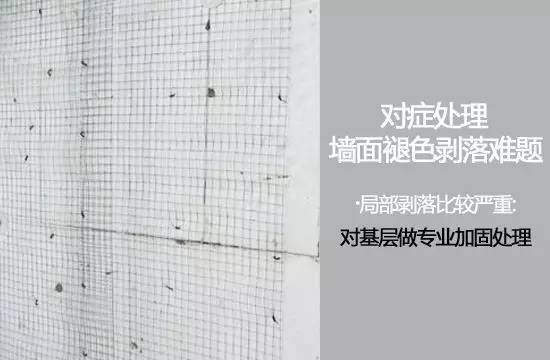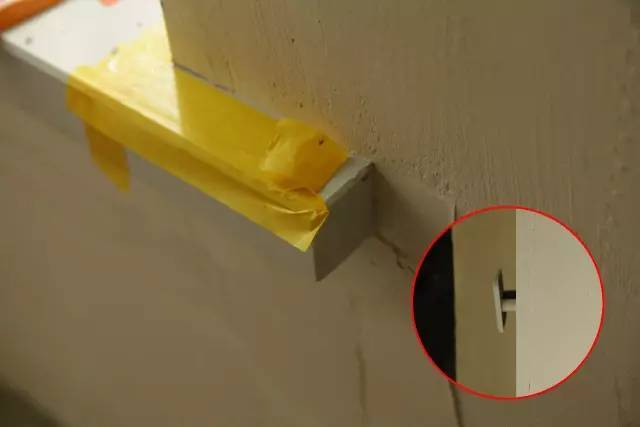|

# 高温天墙面处理,先来看看这份实用秘籍!

气温持续升高、潮湿多雨，对正在施工或是装修后不久的墙面，都会出现不同程度的问题。考虑到江浙沪地区夏季高温、多雨的天气情况，墙面施工一定要注意细节上的处理，进而保证施工质量！▲墙面处理与至关重要的气温条件：仔细观察，会发现墙体结构的分层很明显。通常家中常见的墙面问题，如发霉现象，很容易滋生细菌，危害身体健康。也可能是出现了开裂，而且不知道什么时候就会掉下来，极其不美观。因此，趁手的工具非常重要。▲墙面基层处理工具：墙面在涂刷墙面漆和铺贴壁纸前，都需要提前对墙面基层进行处理，包括整平和找平。墙面粉化、掉皮、褪色是最常见也是最难处理的墙面问题，不同原因引致的的墙面问题其解决方法不一而足，且不能一味采用同样的方法，否则很有可能事倍功半。▲墙面开槽工具：这类工具种类较多，其中水电开槽机是比较专业的，可一次性开出不同角度、宽度、深度的线槽，且无需辅助工具。此外，开槽也可用电钻开槽，只不过开的槽一般比较粗糙。▲墙面涂刷工具 ：通常情况下，家装中常用的墙面涂刷方法有三种：刷涂、滚涂、喷涂。装修的时候，在衡量各种涂刷方法的优劣后，根据自己的喜好选择最合适的涂刷方法。从而选择相应的涂刷工具。 此外，墙面刷漆施工过程中，比较常用到的还有分色纸和砂纸。▲铲除剥落部分重刮腻子：白墙或者裸墙局部起鼓或者轻微龟裂剥落的话，可以把出现问题的地方铲除，并将铲除部分的边缘磨平滑后，重新刮一次腻子，上底漆，再刷再涂，不过可能会跟原来的墙面有一点色差。▲对基层做专业加固处理：对于局部剥落比较严重的情况，就有必要考量是否墙面基底根本没做好了。将原墙体铲除至墙面基层，用界面剂或者封固底漆重新涂刷一遍，再按照粉刷石膏找平、贴网格布、批刮墙衬3遍后打磨平整，整个基层质量此时就会好很多。▲直接滚刷面漆覆盖局部：如果是简单的掉色，建议采取滚刷的方式，覆盖新一轮底漆和相似的面漆就可以，需先判断墙面的腻子层此时的质量：选墙面掉色最坏的一片用水湿透后用铲子用力产铲，若无粉化或脱落现，可直接用细砂纸打磨平整处理干净后刷上新底漆和面漆即可。▲拆除旧墙全面施工刷新：全面施工等于重新装修一样，工程量是最大的，适用于存在多处开裂、掉皮、起粉的墙面。首先墙面要全部铲除至水泥层，若是年代久未装修的房子，更是需要铲除到砖墙体表面，然后全面施工翻新墙面。▲高温环境对墙面基层的影响：按照今年夏季的天气情况来看，高温干燥天气居多。而涂料适宜的施工湿度为60~70，所以说，较低的湿度有利于涂料成膜，从而提高施工进度，但如果湿度太低，空气太干燥，溶剂型涂料溶剂挥发过快，水乳型涂料干燥也快，均会使结膜不够完全，因此也不宜施工。▲阳光强烈、也就是紫外线辐射强：基层表面温度太高，会导致水或溶剂挥发过快，使得不要成膜不良，影响涂层质量。除了干燥和阳光暴晒，大风会加速溶剂或水分的蒸发过程，使成膜不良，与油漆工的舒适程度相比，晴朗的天气状况对涂装工程更重要，恶劣的涂装条件将使涂装工程大打折扣。▲完成抹灰层工艺后，涂料干燥、结膜需要在一定温度、湿度条件下进行：不同类型的涂料有其最佳的成膜条件，通常溶剂型涂料宜在5~30℃气温条件下施工，水乳型涂料宜在10~35℃气温条件下施工。而夏季过热的天气会使涂料干燥过快，并使涂料耐久性受到影响▲相比新房，老房墙面处理的难度更大：破坏严重的话，只有铲至墙基重新粉刷。如果不对症下药，最终的效果很难令人满意。用铲子对基层进行处理，清除松动空鼓部位，局部修补的效果肯定不会非常理想，如果想焕然一新，建议全部铲除。用刷子清除墙面灰尘，对于较大的缝隙，用腻子填补结实，配合胶水使用，这样批腻子不会起泡，干燥后打磨光滑。

`声明：本文由入驻焦点开放平台的作者撰写，除焦点官方账号外，观点仅代表作者本人，不代表焦点立场错误信息举报电话： 400-099-0099，邮箱：jubao@vip.sohu.com，或点此进行意见反馈，或点此进行举报投诉。`A B C D E F G H J K L M N P Q R S T W X Y Z
A - B - C - D - E
• A
• 鞍山
• 安庆
• 安阳
• 安顺
• 安康
• 澳门
• B
• 北京
• 保定
• 包头
• 巴彦淖尔
• 本溪
• 蚌埠
• 亳州
• 滨州
• 北海
• 百色
• 巴中
• 毕节
• 保山
• 宝鸡
• 白银
• 巴州
• C
• 承德
• 沧州
• 长治
• 赤峰
• 朝阳
• 长春
• 常州
• 滁州
• 池州
• 长沙
• 常德
• 郴州
• 潮州
• 崇左
• 重庆
• 成都
• 楚雄
• 昌都
• 慈溪
• 常熟
• D
• 大同
• 大连
• 丹东
• 大庆
• 东营
• 德州
• 东莞
• 德阳
• 达州
• 大理
• 德宏
• 定西
• 儋州
• 东平
• E
• 鄂尔多斯
• 鄂州
• 恩施
F - G - H - I - J
• F
• 抚顺
• 阜新
• 阜阳
• 福州
• 抚州
• 佛山
• 防城港
• G
• 赣州
• 广州
• 桂林
• 贵港
• 广元
• 广安
• 贵阳
• 固原
• H
• 邯郸
• 衡水
• 呼和浩特
• 呼伦贝尔
• 葫芦岛
• 哈尔滨
• 黑河
• 淮安
• 杭州
• 湖州
• 合肥
• 淮南
• 淮北
• 黄山
• 菏泽
• 鹤壁
• 黄石
• 黄冈
• 衡阳
• 怀化
• 惠州
• 河源
• 贺州
• 河池
• 海口
• 红河
• 汉中
• 海东
• 怀来
• I
• J
• 晋中
• 锦州
• 吉林
• 鸡西
• 佳木斯
• 嘉兴
• 金华
• 景德镇
• 九江
• 吉安
• 济南
• 济宁
• 焦作
• 荆门
• 荆州
• 江门
• 揭阳
• 金昌
• 酒泉
• 嘉峪关
K - L - M - N - P
• K
• 开封
• 昆明
• 昆山
• L
• 廊坊
• 临汾
• 辽阳
• 连云港
• 丽水
• 六安
• 龙岩
• 莱芜
• 临沂
• 聊城
• 洛阳
• 漯河
• 娄底
• 柳州
• 来宾
• 泸州
• 乐山
• 六盘水
• 丽江
• 临沧
• 拉萨
• 林芝
• 兰州
• 陇南
• M
• 牡丹江
• 马鞍山
• 茂名
• 梅州
• 绵阳
• 眉山
• N
• 南京
• 南通
• 宁波
• 南平
• 宁德
• 南昌
• 南阳
• 南宁
• 内江
• 南充
• P
• 盘锦
• 莆田
• 平顶山
• 濮阳
• 攀枝花
• 普洱
• 平凉
Q - R - S - T - W
• Q
• 秦皇岛
• 齐齐哈尔
• 衢州
• 泉州
• 青岛
• 清远
• 钦州
• 黔南
• 曲靖
• 庆阳
• R
• 日照
• 日喀则
• S
• 石家庄
• 沈阳
• 双鸭山
• 绥化
• 上海
• 苏州
• 宿迁
• 绍兴
• 宿州
• 三明
• 上饶
• 三门峡
• 商丘
• 十堰
• 随州
• 邵阳
• 韶关
• 深圳
• 汕头
• 汕尾
• 三亚
• 三沙
• 遂宁
• 山南
• 商洛
• 石嘴山
• T
• 天津
• 唐山
• 太原
• 通辽
• 铁岭
• 泰州
• 台州
• 铜陵
• 泰安
• 铜仁
• 铜川
• 天水
• 天门
• W
• 乌海
• 乌兰察布
• 无锡
• 温州
• 芜湖
• 潍坊
• 威海
• 武汉
• 梧州
• 渭南
• 武威
• 吴忠
• 乌鲁木齐
X - Y - Z
• X
• 邢台
• 徐州
• 宣城
• 厦门
• 新乡
• 许昌
• 信阳
• 襄阳
• 孝感
• 咸宁
• 湘潭
• 湘西
• 西双版纳
• 西安
• 咸阳
• 西宁
• 仙桃
• 西昌
• Y
• 运城
• 营口
• 盐城
• 扬州
• 鹰潭
• 宜春
• 烟台
• 宜昌
• 岳阳
• 益阳
• 永州
• 阳江
• 云浮
• 玉林
• 宜宾
• 雅安
• 玉溪
• 延安
• 榆林
• 银川
• Z
• 张家口
• 镇江
• 舟山
• 漳州
• 淄博
• 枣庄
• 郑州
• 周口
• 驻马店
• 株洲
• 张家界
• 珠海
• 湛江
• 肇庆
• 中山
• 自贡
• 资阳
• 遵义
• 昭通
• 张掖
• 中卫

1室1厅1厨1卫1阳台

1
2
3
4
5

0
1
2

1

1

0
1
2
3报名成功，资料已提交审核A B C D E F G H J K L M N P Q R S T W X Y Z
A - B - C - D - E
• A
• 鞍山
• 安庆
• 安阳
• 安顺
• 安康
• 澳门
• B
• 北京
• 保定
• 包头
• 巴彦淖尔
• 本溪
• 蚌埠
• 亳州
• 滨州
• 北海
• 百色
• 巴中
• 毕节
• 保山
• 宝鸡
• 白银
• 巴州
• C
• 承德
• 沧州
• 长治
• 赤峰
• 朝阳
• 长春
• 常州
• 滁州
• 池州
• 长沙
• 常德
• 郴州
• 潮州
• 崇左
• 重庆
• 成都
• 楚雄
• 昌都
• 慈溪
• 常熟
• D
• 大同
• 大连
• 丹东
• 大庆
• 东营
• 德州
• 东莞
• 德阳
• 达州
• 大理
• 德宏
• 定西
• 儋州
• 东平
• E
• 鄂尔多斯
• 鄂州
• 恩施
F - G - H - I - J
• F
• 抚顺
• 阜新
• 阜阳
• 福州
• 抚州
• 佛山
• 防城港
• G
• 赣州
• 广州
• 桂林
• 贵港
• 广元
• 广安
• 贵阳
• 固原
• H
• 邯郸
• 衡水
• 呼和浩特
• 呼伦贝尔
• 葫芦岛
• 哈尔滨
• 黑河
• 淮安
• 杭州
• 湖州
• 合肥
• 淮南
• 淮北
• 黄山
• 菏泽
• 鹤壁
• 黄石
• 黄冈
• 衡阳
• 怀化
• 惠州
• 河源
• 贺州
• 河池
• 海口
• 红河
• 汉中
• 海东
• 怀来
• I
• J
• 晋中
• 锦州
• 吉林
• 鸡西
• 佳木斯
• 嘉兴
• 金华
• 景德镇
• 九江
• 吉安
• 济南
• 济宁
• 焦作
• 荆门
• 荆州
• 江门
• 揭阳
• 金昌
• 酒泉
• 嘉峪关
K - L - M - N - P
• K
• 开封
• 昆明
• 昆山
• L
• 廊坊
• 临汾
• 辽阳
• 连云港
• 丽水
• 六安
• 龙岩
• 莱芜
• 临沂
• 聊城
• 洛阳
• 漯河
• 娄底
• 柳州
• 来宾
• 泸州
• 乐山
• 六盘水
• 丽江
• 临沧
• 拉萨
• 林芝
• 兰州
• 陇南
• M
• 牡丹江
• 马鞍山
• 茂名
• 梅州
• 绵阳
• 眉山
• N
• 南京
• 南通
• 宁波
• 南平
• 宁德
• 南昌
• 南阳
• 南宁
• 内江
• 南充
• P
• 盘锦
• 莆田
• 平顶山
• 濮阳
• 攀枝花
• 普洱
• 平凉
Q - R - S - T - W
• Q
• 秦皇岛
• 齐齐哈尔
• 衢州
• 泉州
• 青岛
• 清远
• 钦州
• 黔南
• 曲靖
• 庆阳
• R
• 日照
• 日喀则
• S
• 石家庄
• 沈阳
• 双鸭山
• 绥化
• 上海
• 苏州
• 宿迁
• 绍兴
• 宿州
• 三明
• 上饶
• 三门峡
• 商丘
• 十堰
• 随州
• 邵阳
• 韶关
• 深圳
• 汕头
• 汕尾
• 三亚
• 三沙
• 遂宁
• 山南
• 商洛
• 石嘴山
• T
• 天津
• 唐山
• 太原
• 通辽
• 铁岭
• 泰州
• 台州
• 铜陵
• 泰安
• 铜仁
• 铜川
• 天水
• 天门
• W
• 乌海
• 乌兰察布
• 无锡
• 温州
• 芜湖
• 潍坊
• 威海
• 武汉
• 梧州
• 渭南
• 武威
• 吴忠
• 乌鲁木齐
X - Y - Z
• X
• 邢台
• 徐州
• 宣城
• 厦门
• 新乡
• 许昌
• 信阳
• 襄阳
• 孝感
• 咸宁
• 湘潭
• 湘西
• 西双版纳
• 西安
• 咸阳
• 西宁
• 仙桃
• 西昌
• Y
• 运城
• 营口
• 盐城
• 扬州
• 鹰潭
• 宜春
• 烟台
• 宜昌
• 岳阳
• 益阳
• 永州
• 阳江
• 云浮
• 玉林
• 宜宾
• 雅安
• 玉溪
• 延安
• 榆林
• 银川
• Z
• 张家口
• 镇江
• 舟山
• 漳州
• 淄博
• 枣庄
• 郑州
• 周口
• 驻马店
• 株洲
• 张家界
• 珠海
• 湛江
• 肇庆
• 中山
• 自贡
• 资阳
• 遵义
• 昭通
• 张掖
• 中卫• 手机• 分享
• 设计
免费设计
• 计算器
装修计算器
• 入驻
合作入驻
• 联系
联系我们
• 置顶
返回顶部